@coder-pig 2018-04-17T13:23:38.000000Z 字数 10970 阅读 1703

# 小猪的Python学习之旅 —— 16.再尝Python数据分析：采集拉勾网数据分析Android就业行情

Python## 1.知道下数据分析三件套

：《Python for Data Analysis, 2nd Edition》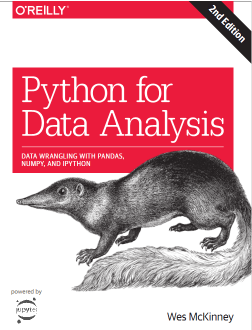### numpy库：

NumPy是在在一个连续的内存块中存储数据，独立于其他Python内置对象。
NumPy的C语言编写的算法库可以操作内存，而不必进行类型检查或其它

### pandas库：

DataFrame (面向列的二维表结构)

## 2.数据爬取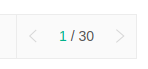F12开发者选项，打开抓包，Network选项卡clear一下，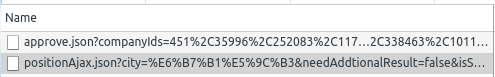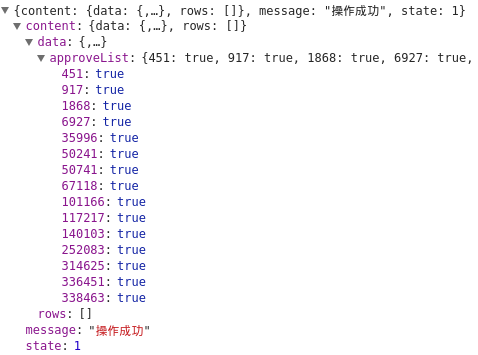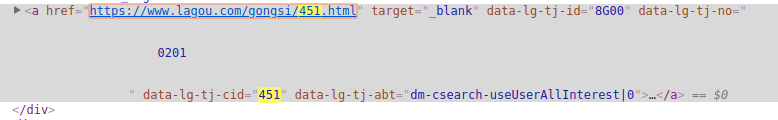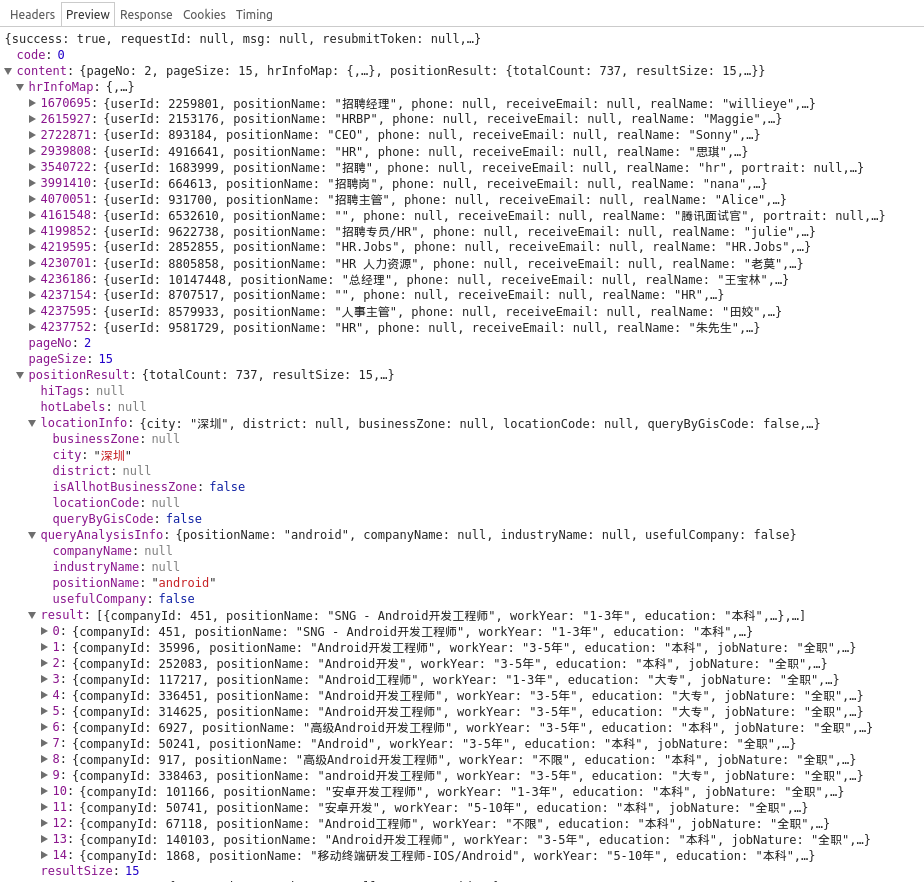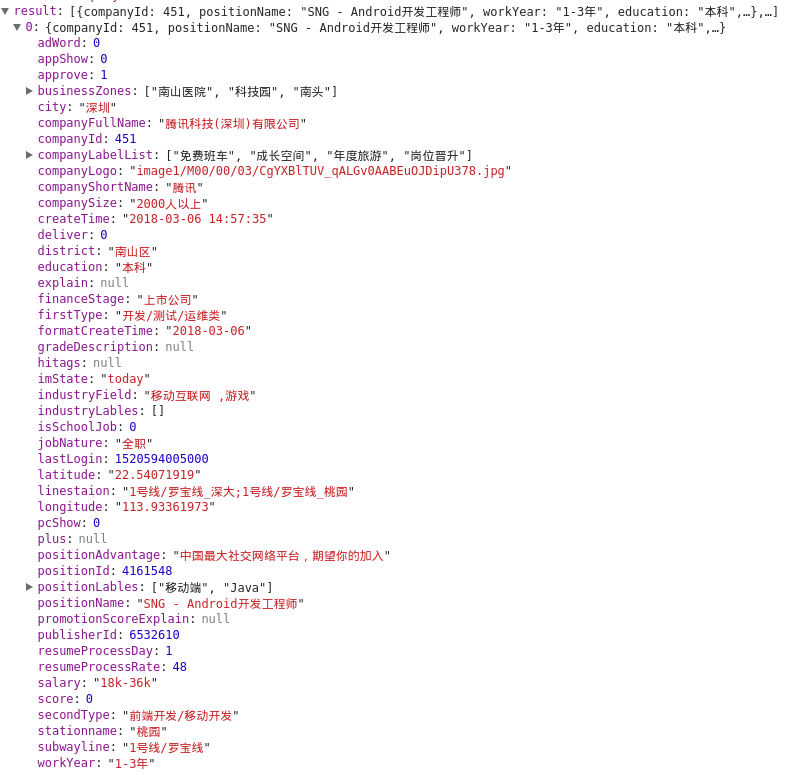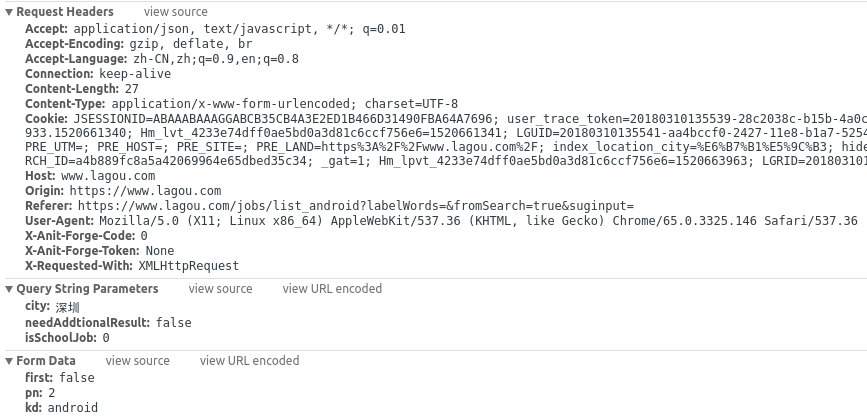• city: 深圳
• isSchoolJob: 0

• first: false
• pn: 2
• kd: android

pn是页码，其他的都不用动！都清楚了，接着就写下代码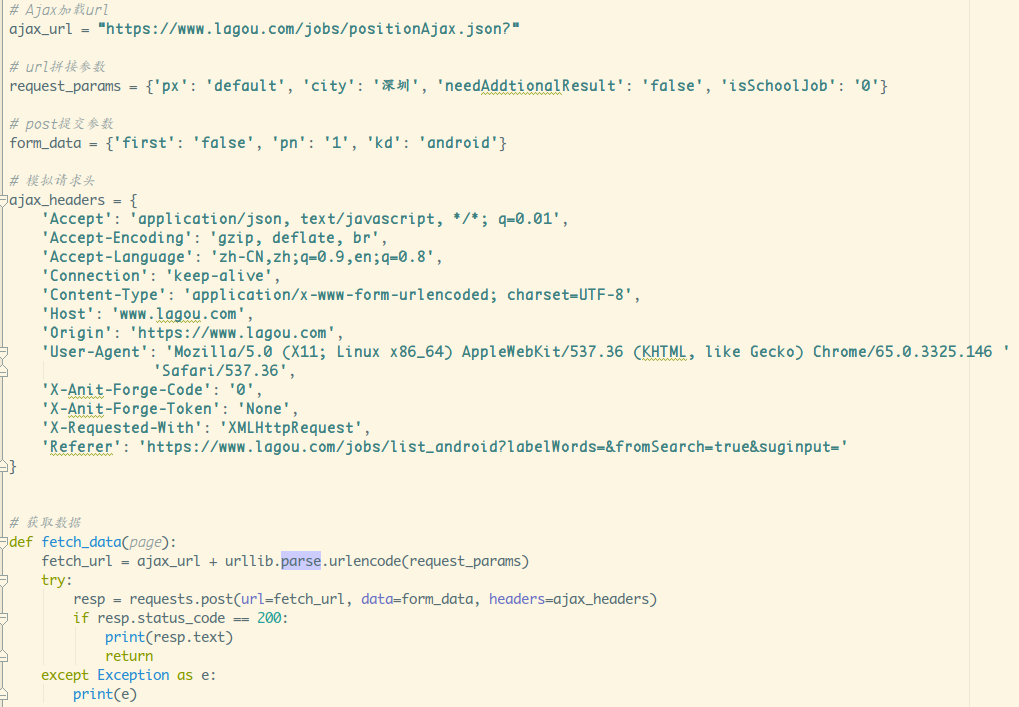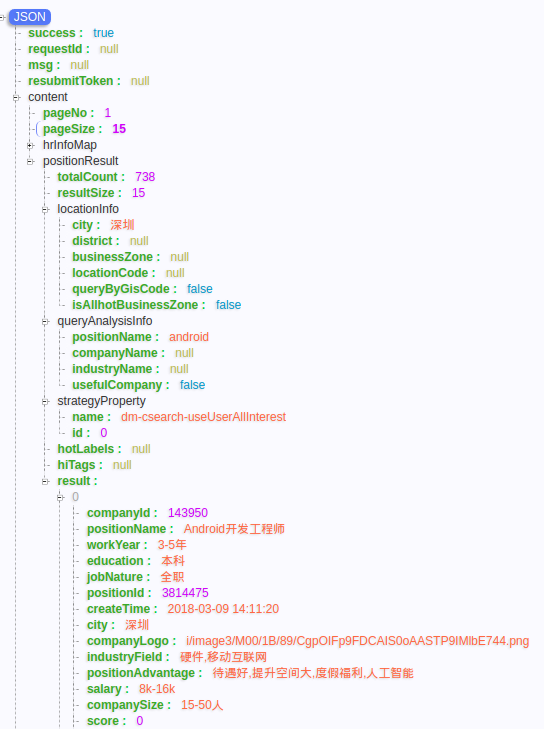公司id招聘岗位id公司全名招聘职位名工作年限
学历性质行业领域公司优势, 薪资范围公司规模
技能标签融资状态公司标签所在区域公司经度
公司纬度

Json结构，我试着写了这样的正则：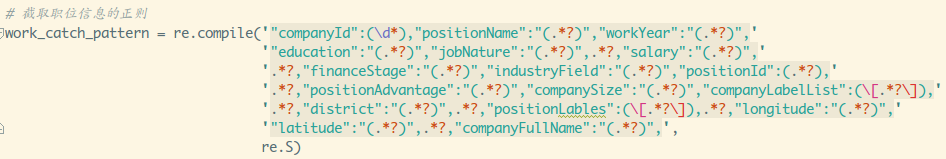## 3.用pandas的to_csv方法把数据都塞Excel里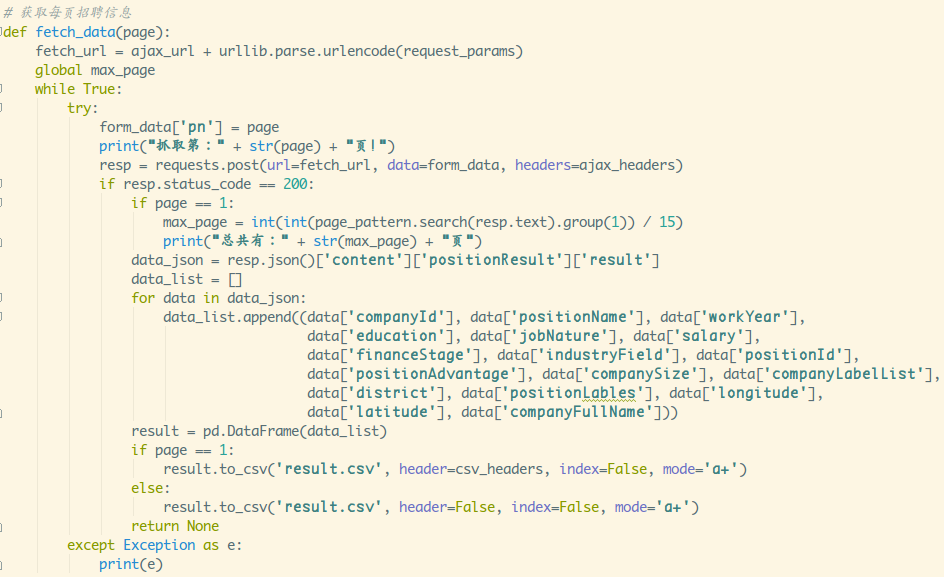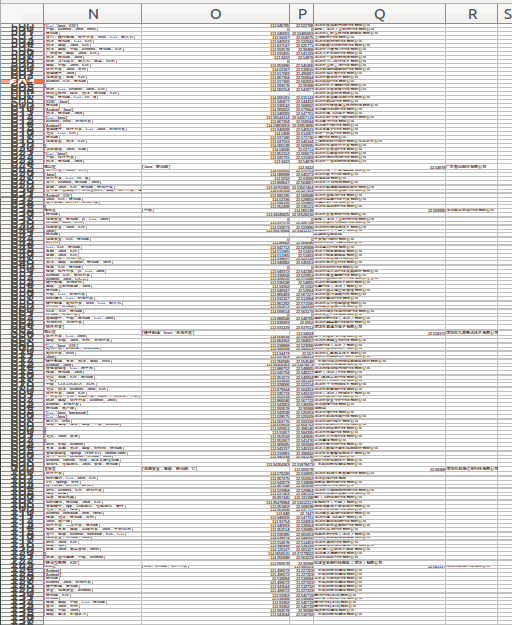Android高级开发工程师&nbsp最新iMAC&nbsp双休&nbsp待遇丰厚&nbspAndroid系统&amp移动开发经理 (Android &amp移动App开发工程师（Android&amp

html.unescape()方法转义一波，对应相反的方法escape()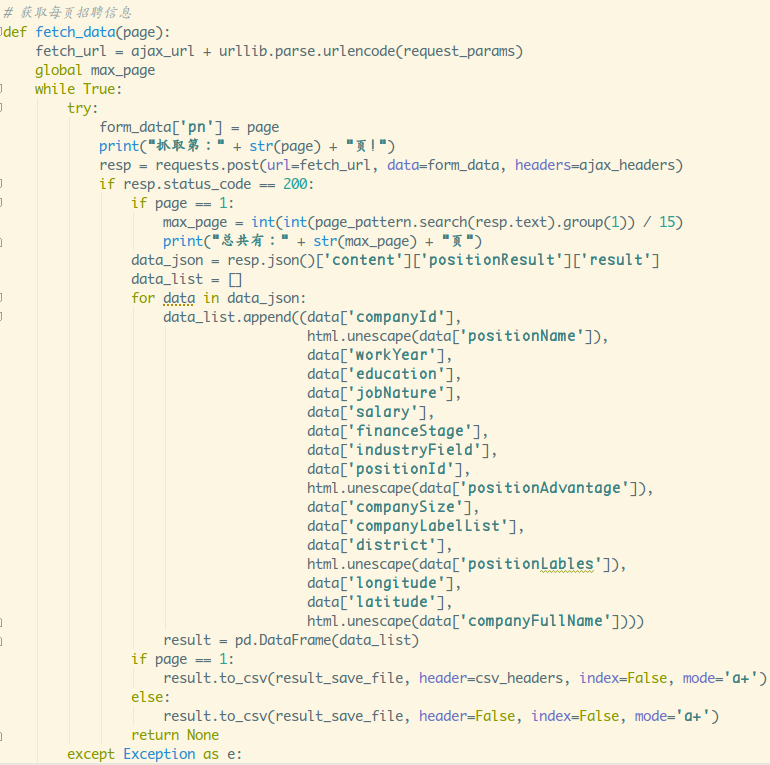## 4.数据分析前可能遇到的一些问题

### 1）matplotlib中文乱码问题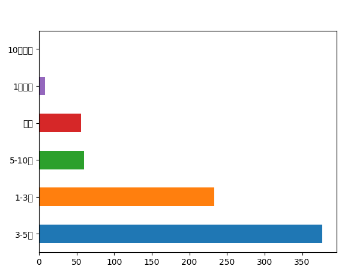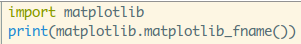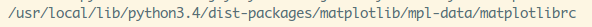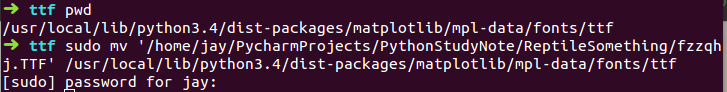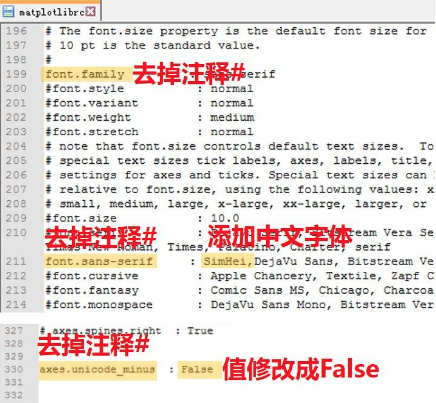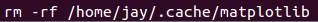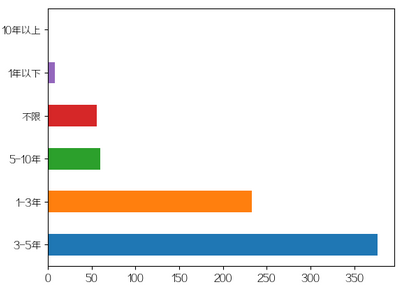### 2）matplotlib绘制显示不全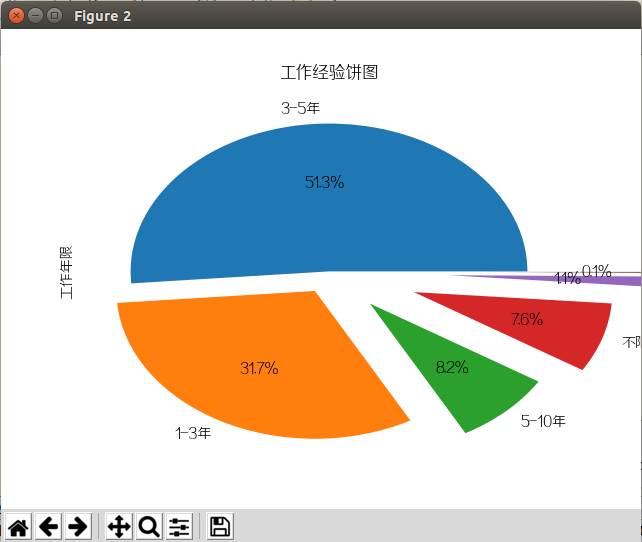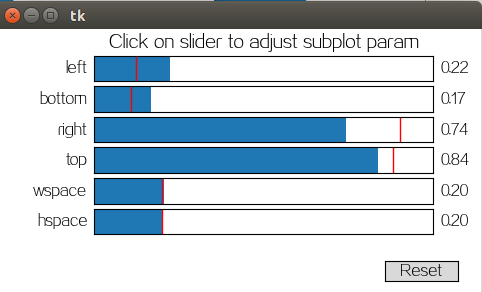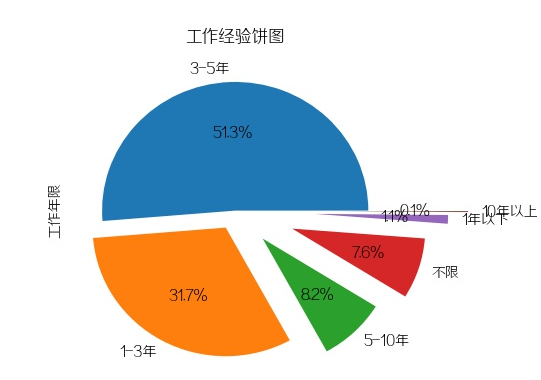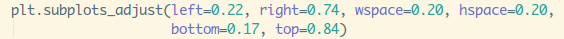## 5.开始数据分析

### 一.分析招聘公司的一些情况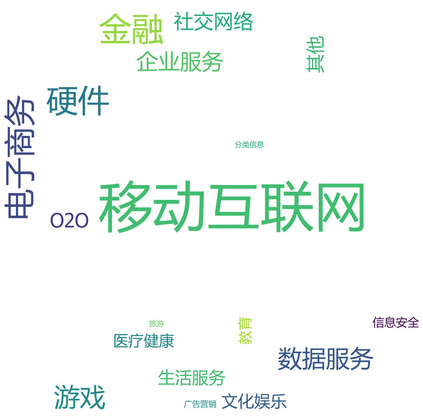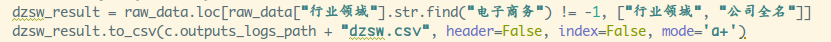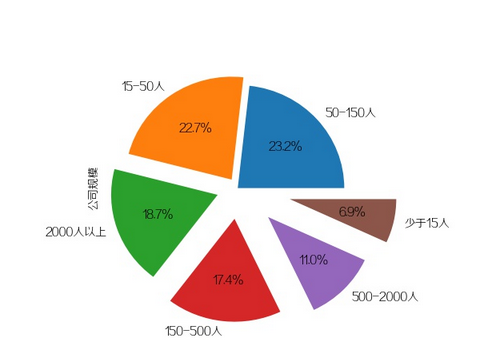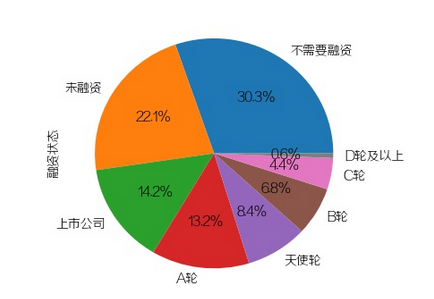PS：卧槽，15-50人和未融资公司的百分比竟然异常接近，应该大部分都是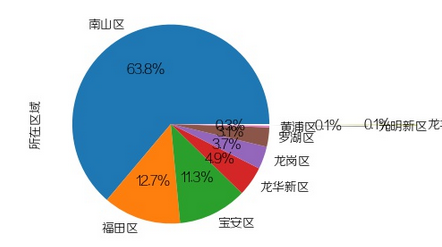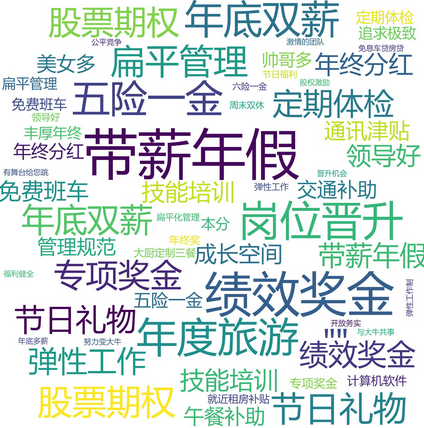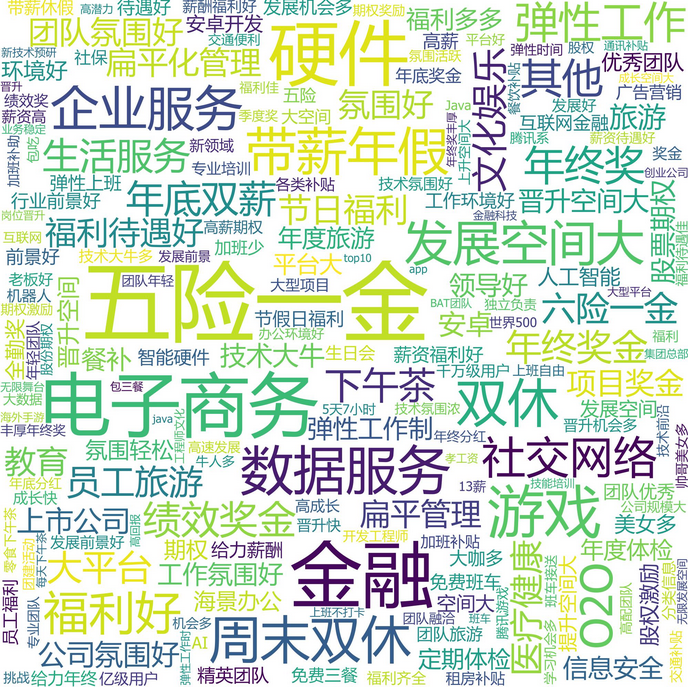### 二.分析对招聘者的一些要求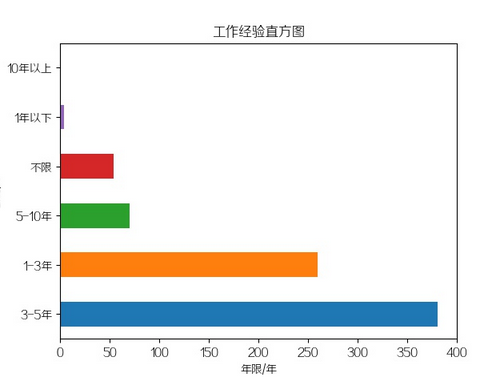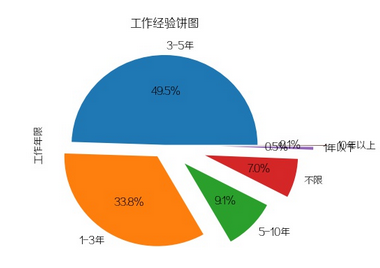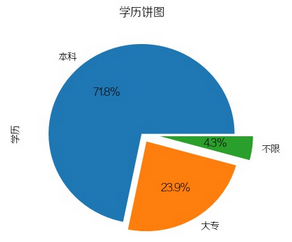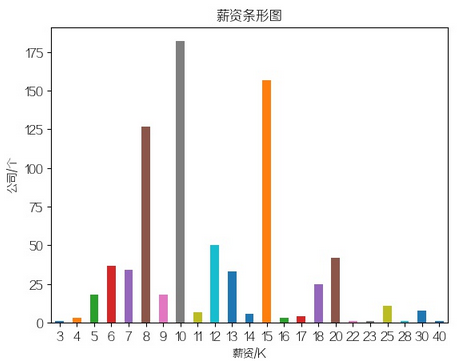10k - 3年经验左右的一般的中初级工程师
15k - 3-5年经验的技术较好的中高级工程师
8k - 1到3年内的初中级工程师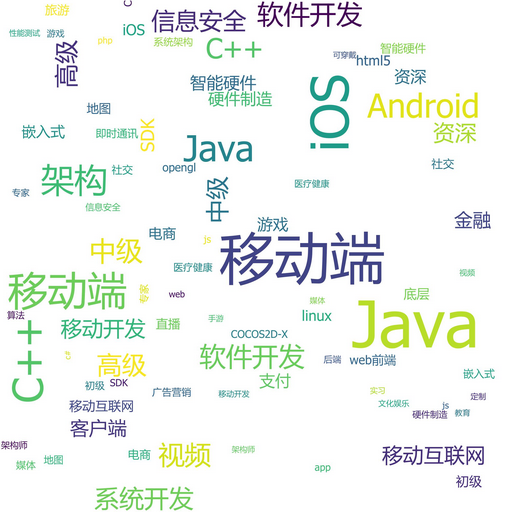Java, iOS，架构，C++，系统开发，中级，高级，资深，视频，信息安全...## 6.小结# 拉勾网Android招聘数据分析import urllib.parseimport requestsimport xlwtimport xlrdimport tools as timport pandas as pdimport geopandas as gpimport reimport randomimport timeimport htmlimport matplotlib.pyplot as pltimport numpy as npfrom wordcloud import WordCloud, STOPWORDS, ImageColorGeneratorfrom collections import Counterfrom scipy.misc import imreadimport config as cfrom shapely.geometry import Point, Polygonmax_page = 1result_save_file = c.outputs_logs_path + 'result.csv'pic_save_path = c.outputs_pictures_path + 'LaGou/'default_font = c.res_documents + 'wryh.ttf'  # 生成词云用的默认字体default_mask = c.res_pictures + 'default_mask.jpg'  # 默认遮罩图片# Ajax加载urlajax_url = "https://www.lagou.com/jobs/positionAjax.json?"# url拼接参数request_params = {'px': 'default', 'city': '深圳', 'needAddtionalResult': 'false', 'isSchoolJob': '0'}# post提交参数form_data = {'first': 'false', 'pn': '1', 'kd': 'android'}# 获得页数的正则page_pattern = re.compile('"totalCount":(\d*),', re.S)# csv表头csv_headers = [    '公司id', '职位名称', '工作年限', '学历', '职位性质', '薪资',    '融资状态', '行业领域', '招聘岗位id', '公司优势', '公司规模',    '公司标签', '所在区域', '技能标签', '公司经度', '公司纬度', '公司全名']# 模拟请求头ajax_headers = {    'Accept': 'application/json, text/javascript, */*; q=0.01',    'Accept-Encoding': 'gzip, deflate, br',    'Accept-Language': 'zh-CN,zh;q=0.9,en;q=0.8',    'Connection': 'keep-alive',    'Content-Type': 'application/x-www-form-urlencoded; charset=UTF-8',    'Host': 'www.lagou.com',    'Origin': 'https://www.lagou.com',    'User-Agent': 'Mozilla/5.0 (X11; Linux x86_64) AppleWebKit/537.36 (KHTML, like Gecko) Chrome/65.0.3325.146 '                  'Safari/537.36',    'X-Anit-Forge-Code': '0',    'X-Anit-Forge-Token': 'None',    'X-Requested-With': 'XMLHttpRequest',    'Referer': 'https://www.lagou.com/jobs/list_android?labelWords=&fromSearch=true&suginput='}# 获取每页招聘信息def fetch_data(page):    fetch_url = ajax_url + urllib.parse.urlencode(request_params)    global max_page    while True:        try:            form_data['pn'] = page            print("抓取第：" + str(page) + "页!")            resp = requests.post(url=fetch_url, data=form_data, headers=ajax_headers)            if resp.status_code == 200:                if page == 1:                    max_page = int(int(page_pattern.search(resp.text).group(1)) / 15)                    print("总共有：" + str(max_page) + "页")                data_json = resp.json()['content']['positionResult']['result']                data_list = []                for data in data_json:                    data_list.append((data['companyId'],                                      html.unescape(data['positionName']),                                      data['workYear'],                                      data['education'],                                      data['jobNature'],                                      data['salary'],                                      data['financeStage'],                                      data['industryField'],                                      data['positionId'],                                      html.unescape(data['positionAdvantage']),                                      data['companySize'],                                      data['companyLabelList'],                                      data['district'],                                      html.unescape(data['positionLables']),                                      data['longitude'],                                      data['latitude'],                                      html.unescape(data['companyFullName'])))                    result = pd.DataFrame(data_list)                if page == 1:                    result.to_csv(result_save_file, header=csv_headers, index=False, mode='a+')                else:                    result.to_csv(result_save_file, header=False, index=False, mode='a+')                return None        except Exception as e:            print(e)# 生成词云文件def make_wc(content, file_name, mask_pic=default_mask, font=default_font):    bg_pic = imread(mask_pic)    pic_colors = ImageColorGenerator(bg_pic)    wc = WordCloud(font_path=font, background_color='white', margin=2, max_font_size=250,                   width=2000, height=2000,                   min_font_size=30, max_words=1000)    wc.generate_from_frequencies(content)    wc.to_file(file_name)# 数据分析方法(生成相关文件)def data_analysis(data):    # 1.分析招聘公司的相关信息    # 行业领域    industry_field_list = []    for industry_field in data['行业领域']:        for field in industry_field.strip().replace(" ", ",").replace("、", ",").split(','):            industry_field_list.append(field)    counter = dict(Counter(industry_field_list))    counter.pop('')    make_wc(counter, pic_save_path + "wc_1.jpg")    # 公司规模    plt.figure(1)    data['公司规模'].value_counts().plot(kind='pie', autopct='%1.1f%%', explode=np.linspace(0, 0.5, 6))    plt.subplots_adjust(left=0.22, right=0.74, wspace=0.20, hspace=0.20,                        bottom=0.17, top=0.84)    plt.savefig(pic_save_path + 'result_1.jpg')    plt.close(1)    # 融资状态    plt.figure(2)    data['融资状态'].value_counts().plot(kind='pie', autopct='%1.1f%%')    plt.subplots_adjust(left=0.22, right=0.74, wspace=0.20, hspace=0.20,                        bottom=0.17, top=0.84)    plt.savefig(pic_save_path + 'result_2.jpg')    plt.close(2)    # 所在区域    plt.figure(3)    data['所在区域'].value_counts().plot(kind='pie', autopct='%1.1f%%', explode=[0, 0, 0, 0, 0, 0, 0, 1, 1.5])    plt.subplots_adjust(left=0.31, right=0.74, wspace=0.20, hspace=0.20,                        bottom=0.26, top=0.84)    plt.savefig(pic_save_path + 'result_3.jpg')    plt.close(3)    # 公司标签    tags_list = []    for tags in data['公司标签']:        for tag in tags.strip().replace("[", "").replace("]", "").replace("'", "").split(','):            tags_list.append(tag)    counter = dict(Counter(tags_list))    counter.pop('')    make_wc(counter, pic_save_path + "wc_2.jpg")    # 公司优势    advantage_list = []    for advantage_field in data['公司优势']:        for field in advantage_field.strip().replace(" ", ",").replace("、", ",").replace("，", ",").replace("+", ",") \                .split(','):            industry_field_list.append(field)    counter = dict(Counter(industry_field_list))    counter.pop('')    counter.pop('移动互联网')    make_wc(counter, pic_save_path + "wc_3.jpg")    # 2.分析招聘需求    # 工作年限要求    # 横向条形图    plt.figure(4)    data['工作年限'].value_counts().plot(kind='barh', rot=0)    plt.title("工作经验直方图")    plt.xlabel("年限/年")    plt.ylabel("公司/个")    plt.savefig(pic_save_path + 'result_4.jpg')    plt.close(4)    # 饼图    plt.figure(5)    data['工作年限'].value_counts().plot(kind='pie', autopct='%1.1f%%', explode=np.linspace(0, 0.75, 6))    plt.title("工作经验饼图")    plt.subplots_adjust(left=0.22, right=0.74, wspace=0.20, hspace=0.20,                        bottom=0.17, top=0.84)    plt.savefig(pic_save_path + 'result_5.jpg')    plt.close(5)    # 学历要求    plt.figure(6)    data['学历'].value_counts().plot(kind='pie', autopct='%1.1f%%', explode=(0, 0.1, 0.2))    plt.title("学历饼图")    plt.subplots_adjust(left=0.22, right=0.74, wspace=0.20, hspace=0.20,                        bottom=0.17, top=0.84)    plt.savefig(pic_save_path + 'result_6.jpg')    plt.close(6)    # 薪资(先去掉后部分的最大工资，过滤掉kK以上词汇，获取索引按照整数生序排列)    plt.figure(7)    salary = data['薪资'].str.split('-').str.get(0).str.replace('k|K|以上', "").value_counts()    salary_index = list(salary.index)    salary_index.sort(key=lambda x: int(x))    final_salary = salary.reindex(salary_index)    plt.title("薪资条形图")    final_salary.plot(kind='bar', rot=0)    plt.xlabel("薪资/K")    plt.ylabel("公司/个")    plt.savefig(pic_save_path + 'result_7.jpg')    plt.close(7)    # 技能标签    skill_list = []    for skills in data['技能标签']:        for skill in skills.strip().replace("[", "").replace("]", "").replace("'", "").split(','):            skill_list.append(skill)    counter = dict(Counter(skill_list))    counter.pop('')    counter.pop('Android')    make_wc(counter, pic_save_path + "wc_4.jpg")# 处理数据if __name__ == '__main__':    t.is_dir_existed(pic_save_path)    if not t.is_dir_existed(result_save_file, mkdir=False):        fetch_data(1)        for cur_page in range(2, max_page + 1):            # 随缘休息5-15s            time.sleep(random.randint(5, 15))            fetch_data(cur_page)    else:        raw_data = pd.read_csv(result_save_file)        # data_analysis(raw_data)        # 筛选电子商务公司        dzsw_result = raw_data.loc[raw_data["行业领域"].str.find("电子商务") != -1, ["行业领域", "公司全名"]]        dzsw_result.to_csv(c.outputs_logs_path + "dzsw.csv", header=False, index=False, mode='a+')        # 筛选人15-50人的公司        p_num_result = raw_data.loc[raw_data["所在区域"] == "龙华新区", ["所在区域", "公司全名"]]        p_num_result.to_csv(c.outputs_logs_path + "lhxq.csv", header=False, index=False, mode='a+')

PythonpythonpyPy加群交易屁眼 中的一个关键词即可通过；• 私有
• 公开
• 删除